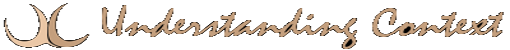# 20 Mar equation

Mathematical formulae or equations are a symbolic form of propositional logic. We must accept the values of the symbols (numbers and variables) and the functions of the operators for the propositions to be well-formed. A formula probably must be well-formed to be correct but while improper structure implies incorrectness, proper structure does not imply correctness.
Keywords: combinatorial explosion
range
quantifiers
proposition
logic
rule
fact
reasoning
form
formalism
References: algorithms
automata
computing
logic
rules

This site uses Akismet to reduce spam. Learn how your comment data is processed.